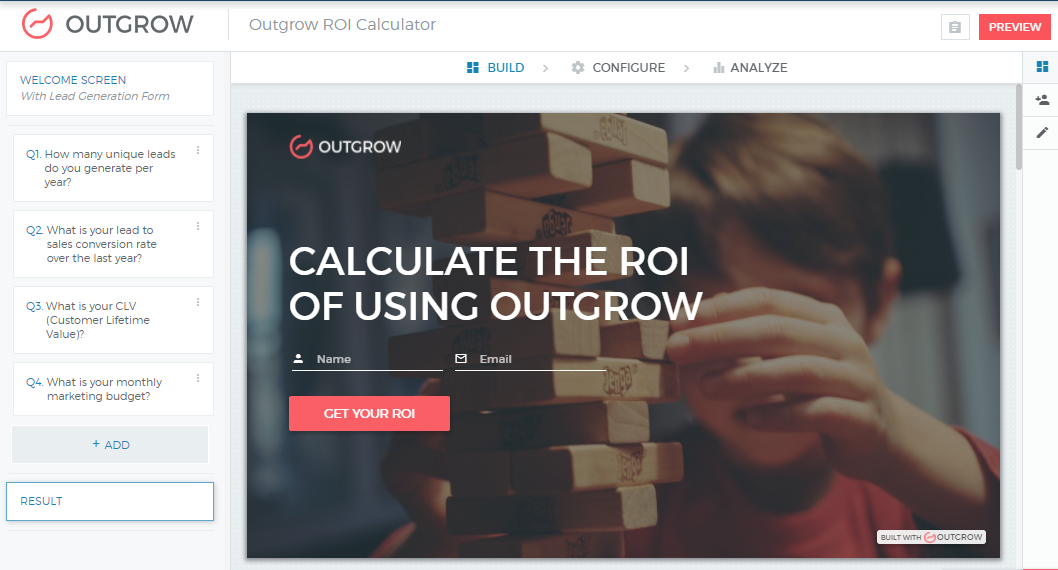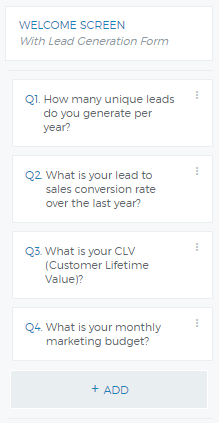## Outgrow Blog

How to build an ROI calculator?
19th October 2016

# How to Build an ROI Calculator?

An RoI calculator usually requires a simple calculation along the lines of:

`RoI (%) = Net Gain (\$) / Total Cost of Investment (\$) * 100`

Note: This can also be written as `RoI = (Total Gain from Investment / Total Investment - 1 ) x 100`.

While a simple calculation, clients usually will not be able to estimate the forwarding-looking Net Gain off the top of their minds. So you will have to structure the calculator questions in a way that the client can easily answer them. Let’s break down this calculation with an example of calculating RoI of buying a subscription to the Outgrow Platform.

The first input we need is the Net Profit or simply put, how much money can one make a net of costs. In formula terms:

`Net Gain (\$) = Marginal Gross Profit from Investment (\$) - Total Investment (\$)`

While total investment is an easy number to discern (since you are the seller you already know that answer), calculating Marginal Gross Profit can be slightly tricky and needs to be broken down further:

`Marginal Gross Profit (\$) = New Customers (#) x Marginal Gross Profit Per Customer (\$)`

Note: Marginal Gross Profit per customer is sometimes referred to as Customer Lifetime Value.

The customer will usually know the revenue per customer but might need help calculating the number of new customers he can get from calculators. That will not be obvious upfront.

`New Customers (#) = Number of New Prospects x Sales Conversion Rate`

For Outgrow, we know that our calculators increase conversions on landing pages by 28% on average. Hence, the number of prospects or leads generated will be 28% more”

`New Customers From Calculators (#) = (Current Number of Prospects x 28%) x Sales Conversion Rate`

The current number of prospects and the sales conversion rates are both numbers that our users should know without any further breakdown. So our final formula break down will be:

`RoI (%) = Net Gain (\$) / Total Investment (\$) x 100`

`RoI (%) = (Marginal Gross Profit from Investment (\$) - Total Investment (\$)) / Total Investment (\$) x 100`

`RoI (%) = ((New Customers from Investment (#) x Marginal Gross Profit Per Customer (\$)) - Total Investment (\$)) / Total Investment (\$) x 100`

`RoI (%) = ((((28% of Current Number of Prospects) x Sales Conversion Rate) x Marginal Gross Profit Per Customer (\$)) - Total Investment (\$)) / Total Investment (\$) x 100`

So the entire calculator requires 3 simple inputs from the user and can calculate RoI.

## How to create a more complex ROI calculator?

Distill the formula to come up with a more accurate forward-looking ROI. Read on.

With data reported through our clients, we know that the sales conversion rate is higher by about 12% when a client comes through a calculator. This is because a sales rep has more information and can target better while a client has more trust in a transparent brand. The result is an increased conversion rate for the salesman and satisfaction for the customer

If we would like to incorporate this case as well, then we just need to add 12% to the original sales conversion rate.

`RoI (%) = ((28% of Current Number of Prospects) x (Original Sales Conversion Rate + 12%) x (Marginal Gross Profit Per Customer (\$)) - Total Investment (\$)) / Total Investment (\$) x 100`

Similarly, you can go deeper and further distill the numbers but we need to keep in mind that these calculators are for marketing and/or for lead generation. So, we need to balance simplicity with accuracy. If we ask too many questions, no doubt we can increase the accuracy but we do run the risk of having a high bounce rate.

Below is an example of how to build a simple ROI calculator on Outgrow –

### 1 – Let us create a calculator on How to calculate ROI of using Outgrow### 2 – Create all the questions most relevant to your calculator. Click on ADD from the left panel and create new questions. You can see we have added 4 questions here.### 3 – Now create a formula in the formula builder.

So to calculate ROI {R1}, you need new leads {R2}, new sales conversation rate {R3} and marketing budget invested in Outgrow {R4}. Remember any percentages in the Outgrow builder will need to be divided by hundred

So on outgrow, the formula will look like –

`RoI (%) = ((0.28 of Current Number of Prospects) x (Original Sales Conversion Rate + 0.12) x (Marginal Gross Profit Per Customer (\$)) - Total Investment (\$)) / Total Investment (\$) x 100`We hope this makes it clear.  You have issues creating this or have no time to build it yourself? We have an awesome customer success team who can build this for. For more details on custom plans, drop us a message by clicking on the chat icon on the bottom right.

The secret to building a viral calculator is simple – but creative yet accurate. How? Take some cues from this guide we curated for you.